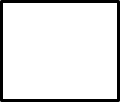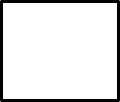1
visibility

Determine the number that makes the equation true.

48 + 4 =– 6

• A

48

• B

58

• C

50

• D

47

Solution:
Consider the given equation.
48 + 4 =– 6

To determine the missing number, first, we need to find: 48 + 4.

Which gives:
48 + 4 = 52

Then,
48 + 4 =– 6

52 =– 6

Next, to find the missing number we find: 52 + 6.

Which gives:
52 + 6 = 58

So,

48 + 4 = 58 – 6

That is, number 58 makes the equation true.